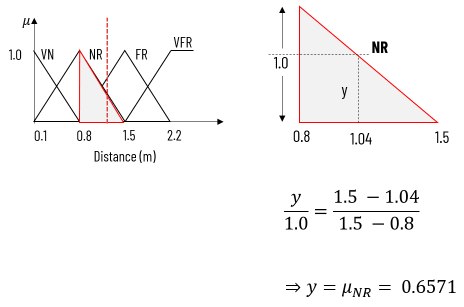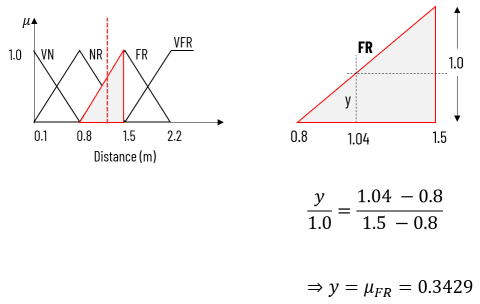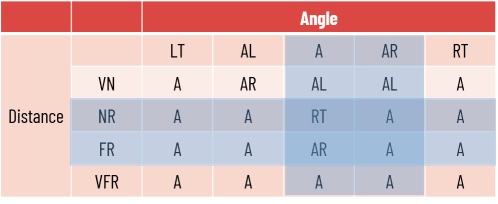Mamdani fuzzy inference method we discussed in previous article, is elaborated here with detailed example.

Example:

Consider that,

D: The distance from the robot to an object

θ: The angle of motion of an object with respect to the robot.

δ: Deviation with respect to D and θ

We assume the range of values of D is [0.1, …. 2.2] in meter and θ is [-90, … , 0, … 90]

Find the deviation of robot from its position for distance D = 1.04 m and angle θ = 30o

Solution:

Database:

The data base (DB) of the FLC are shown below:

Distance::

• VN : Very Near
• NR : Near
• FR : Far
• VFR : Very Far

Angle (angular direction (θ) and deviation (δ)):

• LT : Left
• RT : Right

Graphical representation of fuzzy membership function is shown here for both inputs (distance and direction) and one output (deviation).

Rule base:

Rule base is typically defined by the expert of the domain. For given instance, we are considering following rule base.

Fuzzy linguistic rule : If x is D and y is θ then z is δ

This rule base has 20 IF-THEN rules:

• Rule 1: If (D is VN ) and (θ is LT) Then (δ is A)
• Rule 2: If (D is VN ) and (θ is AL) Then (δ is AR)
• .
• .
• .
• Rule 20: If (D is VF ) and (θ is RT) Then (δ is A)

Fuzzification of inputs:

Given, D = 1.04 m and angle θ = 30o

For this input, we are to decide the deviation δ of the robot as output. First we need to compute the fuzzy values of crisp inputs using following fuzzification method.

As we can see in above diagram, distance D = 1.04 is member of two fuzzy sets called NR and FR.

Similarly, Angle θ = 300 is member of two fuzzy sets called A and AR.

So we need to compute the membership value (i.e. fuzzified value) of both inputs in their corresponding fuzzy sets.

Let us find the membership value for D = 1.04 m in NR and FR fuzzy sets. We can easily find the membership value of any crisp input using similar triangle rule, as shown below:Membership value computation of D = 1.04 m in NR fuzzy setMembership value computation of D = 1.04 m in FR fuzzy set

Similarly, μNR = 0.6571, μFR = 0.3429, μA = 0.3333, μAR = 0.6667

Fired Rules:

Out of 20 rules in rule base, only four rules will fire.

With given set of fuzzy variables, we have four different combination of rules:

• If Distance is NR AND Angle is A Then Deviation is RT
• If Distance is NR AND Angle is AR Then Deviation is A
• If Distance is FR AND Angle is A Then Deviation is AR
• If Distance is FR AND Angle is AR Then Deviation is A

Fired rules are highlighted in following rule base – which is intersection of fuzzy sets of D and θ:Fired rules as intersection of fuzzy sets of D and θ

Computing strength of fired rules:

As we know, μNR = 0.6571, μFR = 0.3429, μA = 0.3333, μAR = 0.6667. Strength (α values) of the fired rules:

α1 = min⁡(μNRA ) = min⁡(0.6571, 0.3333) = 0.3333

α2 = min⁡(μNRAR ) = min⁡(0.6571, 0.6667) = 0.6571

α1 = min⁡(μFRA ) = min⁡(0.3429, 0.3333) = 0.3333

α1 = min⁡(μFRAR ) = min⁡(0.3429, 0.6667) = 0.3429

Strength of fired rules and their corresponding membership in output for each fuzzy rule is shown in following figure.

Aggregation of fuzzy output functions:

To compute the final crisp value of deviation (δ) using mamdani fuzzy inference method, we shall aggregate all fuzzy output functions on same axis, as we did in defuzzification methods.

We will apply various defuzzification method on this aggregated output function to find the final crisp value.

## Watch on YouTube: Mamdani Fuzzy Inference Method

Defuzzification:

Equation to compute are of some standard shapes is shown below:

Area: (ab) / 2

Center: 2a/3

Area: ab

Center: (a1 + a2) / 2

Area: 1/2 (a + b) × h

Center: (b1 + b2) / 2

Center of Sums Method:

Defuzzification using Center of Sums (CoS) method can be derived as follow:

Center of Gravity Method:

Defuzzification using Center of Gravity (CoG) method can be derived as follow:

Area and center of each region is depicted in following figure: